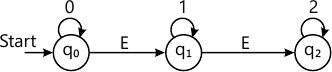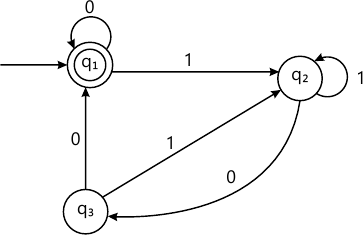MORE IN Theory of Computation
SPPU Computer Engineering (Semester 5)
Theory of Computation
December 2015
Total marks: --
Total time: --
INSTRUCTIONS
(1) Assume appropriate data and state your reasons
(2) Marks are given to the right of every question
(3) Draw neat diagrams wherever necessary

Solve any one question from Q1 and Q2
1 (a) Write regular expressions for the following languages over the alphabet ∑ = {a, b}
i) All strings that do not end with 'aa'.
ii) All strings that contain an even number of 'b' s. iii) All strings which do not contain the substring 'ba'.
6 M
1 (b) Show that for two recursive languages L1 and L2, the language L is also recursive, where L is given by L1 ∩L2.
4 M

2 (a) Consider the following NFA with ∈ - transitions. Find ∈ - closures and then convert this into NFA without ∈ -moves.6 M
2 (b) Prove using mathematical induction the following:
20+21+22+....+2n=2n+1-1, for all integers n≥0.
4 M

Solve any one question from Q3 and Q4
3 (a) Find the regular expression for the set of strings recognized by the given FA. Use Arden?s theorem.6 M
3 (b) Explain, with suitable examples, any two applications of context free grammars.
4 M

4 (a) Convert the following CFG into Chomksy Normal Form (CNF):
S-> AB
A -> CA | ^
B-> DB | ^
C-> 011 | 1
D-> 01
6 M
4 (b) Prove the formula
i) (r * s*)* = (r + s)*.
ii) (ab)* z a* b*.
4 M

Solve any one question from Q5 and Q6
5 (a) Design Turing Machines for each of the following problems:
i) Given two unary numbers, m and n, display,
'G', if m>n, 'E', if m=n, 'L', if m ii) Given two unary numbers, find the Greatest Common Divisor (GCD) of the two numbers.
10 M
5 (b) Justify how a Turing Machine can simulate a General Purpose computer and vice-versa.
8 M

6 (a) Explain : 'The halting problem in Turing Machines is undecidable'.
6 M
6 (b) Design a Turing Machine to perform right shift operation on a binary Number.
6 M
6 (c) Design Post Machine to accept strings that belong to the language L, given by, L = {an b3n |n >= 0}.
6 M

Solve any one question from Q7 and Q8
7 (a) Design PDA for language L={aibjck | i, j, k>1 and i+j=k} that accepts language via
i) Final state.
ii) Empty stack.
10 M
7 (b) Explain the equivalence of PDA with acceptance by final state and empty stack.
6 M

8 (a) Write context free grammar for accepting palindrome strings (even and odd). Also design PDA for the context free grammar.
8 M
8 (b) Consider the PDA with following moves; obtain its equivalent CFG.
((q0, a, Z0) = (q0,aZ0),
(q0, a, a) = (q0, aa),
(q0, b, a) = (q1, ε),
(q1, b, a) = (q1, ε),
(q1, ε, Z0) = (q1, ε)
8 M

Solve any one question from Q9 and Q10
9 (a) What do you mean by Polynomial-time reductions? Describe any problem in detail that is solvable through polynomial time reduction.
8 M
9 (b) What is Satisfiability (SAT) problem? Explain with a suitable example.
8 M

10 (a) Explain the Vertex Cover problem in the context of polynomial-time reductions. Justify with a suitable example.
8 M
10 (b) Explain the following with example.
i) Computational complexity.
ii) 3-SAT problem.
4 M
10 (c) Differentiate between P-class problems and NP-class problems.
4 M

More question papers from Theory of Computation